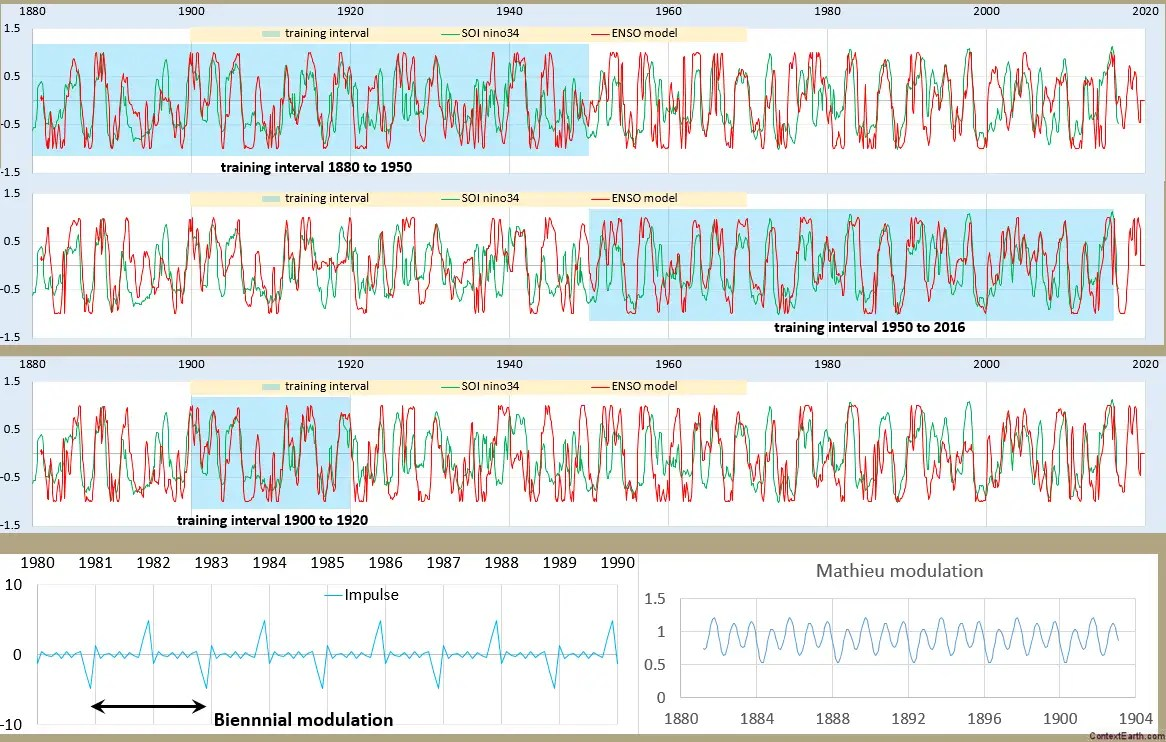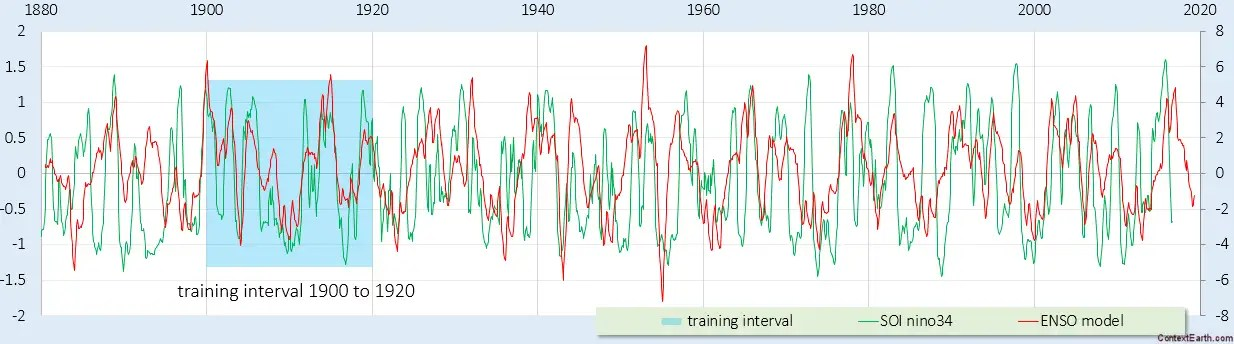# Canonical Solution of Mathieu Equation for ENSO

[mathjax]
From a previous post, we were exploring possible solutions to the Mathieu equation given a pulsed stimulus.  This is a more straightforward decomposition of the differential equation using a spreadsheet.

The Mathieu equation:$f''(t) + omega_0^2 (1 + alpha cos(nu t)) f(t) = F(t)$

can be approximated as a difference equation, where the second derivative f”(t) is ~ (f(t)-2f(t-dt)+f(t-2dt))/dt. But perhaps what we really want is a difference to the previous year and determine if that is enough to reinforce the biennial modulation that we are seeing in the ENSO behavior.

Setting up a spreadsheet with a lag term and a 1-year-prior feedback term, we apply both the biennial impulse-modulated lunar forcing stimulus and a yearly-modulated Mathieu term.Fig. 1: Training (in shaded blue) and test for different intervals.

I was surprised by how remarkable the approximate fit was in the recent post, but this more canonical analysis is even more telling. The number of degrees of freedom in the dozen lunar amplitude terms apparently has no impact on over-fitting, even on the shortest interval in the third chart. There is noise in the ENSO data no doubt, but that noise seems to be secondary considering how the fit seems to mostly capture the real signal. The first two charts are complementary in that regard — the fit is arguably better in each of the training intervals yet the test interval results aren’t really that much different from the direct fit looking at it by eye.

Just like in ocean tidal analysis, the strongest tidal cycles dominate;  in this case the Draconic and Anomalistic monthly, the Draconic and Anomalistic fortnightly, and a Draconic monthly+Anomalistic fortnightly cross term are the strongest (described here). Even though there is much room for weighting these factors differently on orthogonal intervals, the Excel Solver fit hones in on nearly the same weighted set on each interval.  As I said in a previous post, the number of degrees of freedom apparently do not lead to over-fitting issues.

One other feature of this fit was an application of a sin() function applied to the result. This is derived from the Sturm-Liouville solution to Laplace’s tidal equation used in the QBO analysis  — which works effectively to normalize the model to the data, since the correlation coefficient optimizing metric does not scale the result automatically.

Pondering for a moment, perhaps the calculus is not so different to work out after all:

A bottom-line finding is that there is really not much complexity to this unique tidal formulation model of ENSO.  But because of the uniqueness of the seasonal modulation that we apply, it just doesn’t look like the more-or-less regular cycles contributing to sea-level height tidal data.  Essentially similar algorithms are applied to find the right weighting of tidal factors, but whereas the SLH tidal data shows up in daily readings, the ENSO data is year-to-year.

Further, the algorithm does not take more than a minute or two to finish fitting the model to the data. Below is a time lapse of one such trial. Although this isn’t an optimal fit, one can see how the training interval solver adjustment (in the shaded region) pulls the rest of the modeled time-series into alignment with the out-of-band test interval data.# Shortest Training Fit for ENSO

This is remarkable. Using the spreadsheet linked in the last post, the figure below is a model of ENSO derived completely by a training fit over the interval 1900 to 1920, using the Nino3.4 data series and applying the precisely phased Draconic and Anomalistic long-period tidal cycles.Fig. 1 : The ENSO model in red. The blue BG region is used for training of the lunar tidal amplitudes against the Nino3.4 data in green. That data is square root compacted to convert it to an equivalent velocity.

Not much more to say. There is a major disturbance starting in the mid-1980’s, but that is known from a Takens embedding analysis described in the first paper in this post.

# Tidal Model of ENSO

The input forcing to the ENSO model includes combinations of the three major lunar months modulated by the seasonal solar cycle. This makes it conceptually similar to an ocean tidal analysis, but for ENSO we are more concerned about the long-period tides rather than the diurnal and semi-diurnal cycles used in conventional tidal analysis.

The three constituent lunar month factors are:

Month type Length in days
anomalistic 27.554549
tropical 27.321582
draconic 27.212220

So the essential cyclic terms are the following phased sinusoids